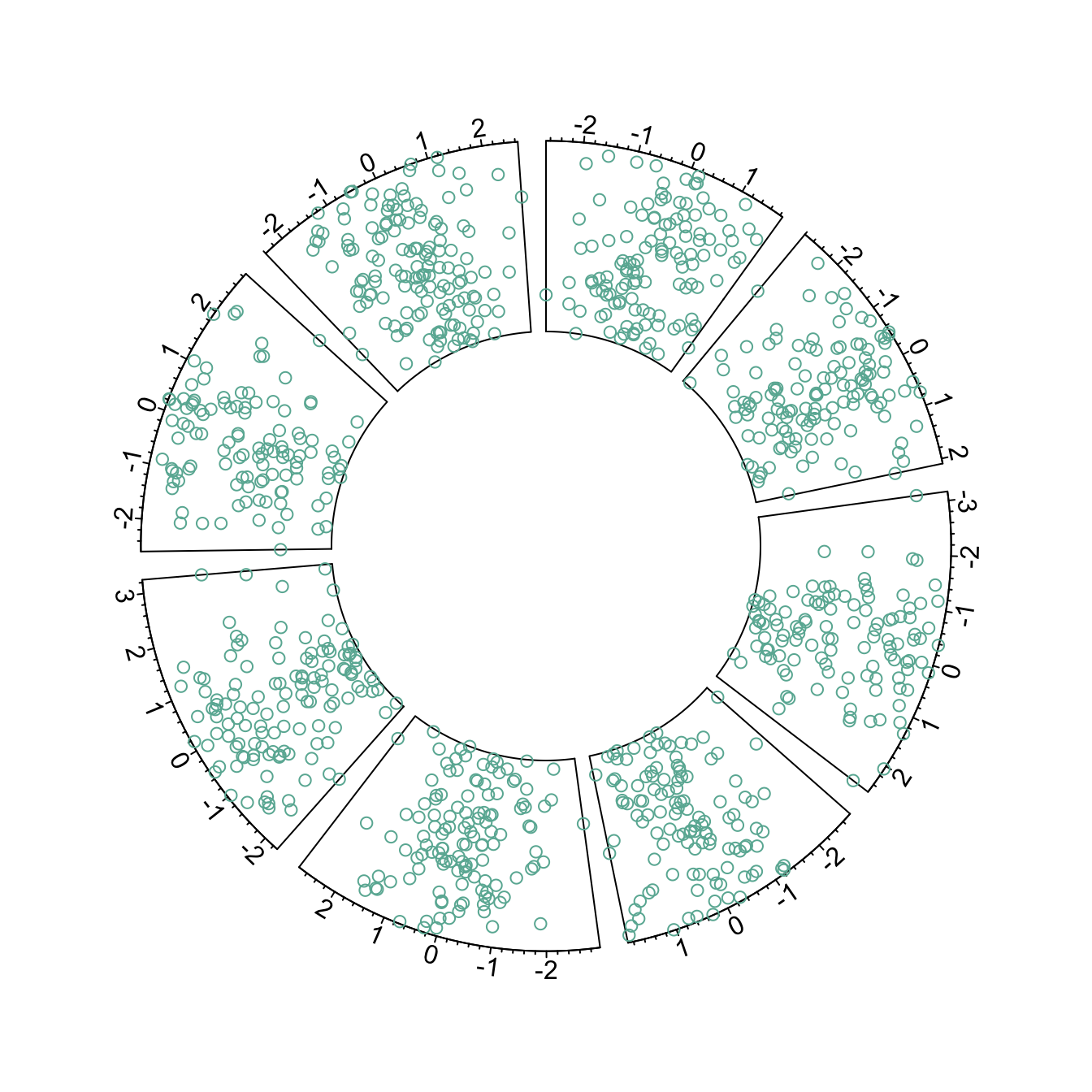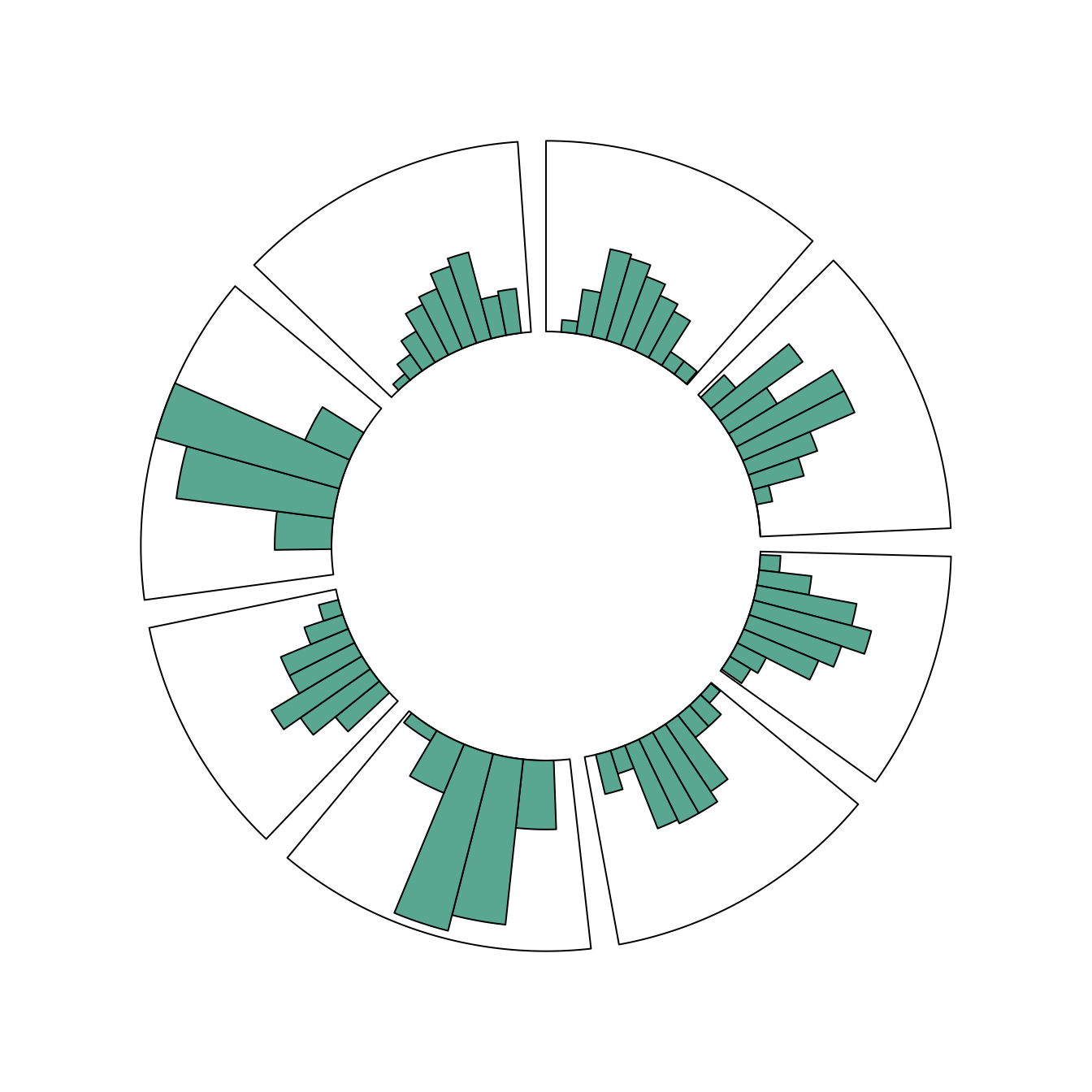# Available circular chart types with circlize

The `circlize` package allows to build circular charts with R. Several chart types are offered: bar, scatter, line, abline and more. This post shows how to build them.

# Circular Scatterplot

Circular scatterplot has already been extensively described in chart #224 and #225.

Here is a reminder:``````# Upload library
library(circlize)
circos.par("track.height" = 0.4)

# Create data
data = data.frame(
factor = sample(letters[1:8], 1000, replace = TRUE),
x = rnorm(1000),
y = runif(1000)
)

# Step1: Initialise the chart giving factor and x-axis.
circos.initialize( factors=data\$factor, x=data\$x )

# Step 2: Build the regions.
circos.trackPlotRegion(factors = data\$factor, y = data\$y, panel.fun = function(x, y) {
circos.axis()
})

circos.trackPoints(data\$factor, data\$x, data\$y, col="#69b3a2")``````

# Circular Line chart

It is possible to switch to line chart using the `circos.trackLines()` function. Visit the line chart section of the gallery to learn how to customize that kind of chart.``````# Upload library
library(circlize)
circos.par("track.height" = 0.4)

# Create data
data = data.frame(
factor = sample(letters[1:8], 1000, replace = TRUE),
x = rnorm(1000),
y = runif(1000)
)

# Step1: Initialise the chart giving factor and x-axis.
circos.initialize( factors=data\$factor, x=data\$x )

# Step 2: Build the regions.
circos.trackPlotRegion(factors = data\$factor, y = data\$y, panel.fun = function(x, y) {
circos.axis()
})

circos.trackLines(data\$factor, data\$x[order(data\$x)], data\$y[order(data\$x)], col = rgb(0.1,0.5,0.8,0.3), lwd=2)``````

# Vertical ablines

The `circos.trackLines()` function can also be used to display vertical ablines.``````# Upload library
library(circlize)
circos.par("track.height" = 0.4)

# Create data
data = data.frame(
factor = sample(letters[1:8], 1000, replace = TRUE),
x = rnorm(1000),
y = runif(1000)
)

# Step1: Initialise the chart giving factor and x-axis.
circos.initialize( factors=data\$factor, x=data\$x )

# Step 2: Build the regions.
circos.trackPlotRegion(factors = data\$factor, y = data\$y, panel.fun = function(x, y) {
circos.axis()
})

circos.trackLines(data\$factor, data\$x[order(data\$x)], data\$y[order(data\$x)], col = rgb(0.1,0.5,0.8,0.3), lwd=2, type="h")``````

# Circular histogram

Note that each plot type must be coherent with what you specified in the `circos.trackPlotRegion` function.

You have to specify an Y axis for a scatterplot as seen before. But not for a histogram that is built with `circos.trackHist()`.``````# Upload library
library(circlize)
circos.par("track.height" = 0.4)

# Create data
data = data.frame(
factor = sample(letters[1:8], 1000, replace = TRUE),
x = rnorm(1000),
y = runif(1000)
)

# Step1: Initialise the chart giving factor and x-axis.
circos.initialize( factors=data\$factor, x=data\$x )

circos.trackHist(data\$factor, data\$x, bg.col = "white", col = "#69b3a2")``````

Related chart types

## Contact

This document is a work by Yan Holtz. Any feedback is highly encouraged. You can fill an issue on Github, drop me a message on Twitter, or send an email pasting yan.holtz.data with gmail.com.